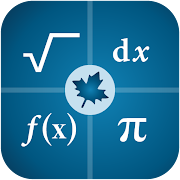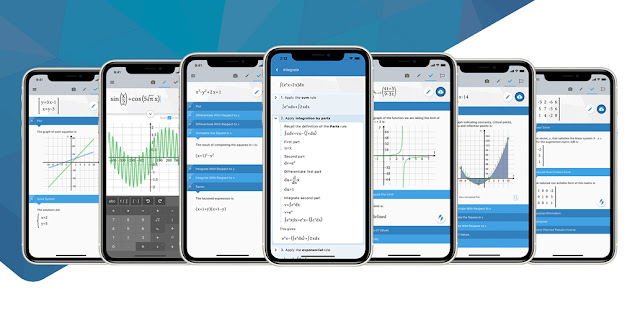Maple Calculator: Math SolverEveryone
6,274
Offers in-app purchasesSolve math problems, visualize graphs of 2-D and 3-D expressions and see step-by-step solutions for a wide variety of high-school and university-level questions.

Maple Calculator is a powerful math solver and versatile math learning tool that gives you answers, 2-D and 3-D graphs, and even step-by-step solutions! Whether you are doing simple calculations or working on university-level math problems, Maple Calculator can do it all. Use it to see the final answer or step-by-step solutions to algebra problems, derivative or integrals, matrix manipulations, differential equations and so much more!

This versatile app makes it incredibly easy to enter, solve, and visualize mathematical problems from algebra, precalculus, calculus, linear algebra, and differential equations, for free! You can even enter problems using your camera, so you can check your homework (& get steps) with a click of a button. This app is your essential companion to help you learn math, whether you are in a classroom or learning from home.

Key Features

• Just click to enter your math: Enter handwritten and typeset math problems at the click of your camera using powerful AI technology. Or, enter your expression into the calculator using the same mathematical notation your teacher uses – your problem is easy to enter because it “looks right”!
• Do all sorts of math: No matter how you enter your problem, you can find derivatives and integrals, factor polynomials, invert matrices, solve systems of equations, solve ODEs, and much more. The app has the power of the world-leading Maple math engine behind it, so it can do a LOT of math!
• Get step-by-step solutions: In addition to the answer, you get fully worked, step-by-step solutions to a wide variety of math problems, including factoring; finding limits, derivatives, and, integrals; performing matrix operations; solving differential equations, and more!.
• Graph problems and results: See 2-D and 3-D graphs of your expression instantly, and watch how the graph changes as you change the expression. You can zoom in, pan, and even rotate 3-D plots to get a closer look at areas of interest.
• Get answers even when you’re offline: Some aspects of the app require an internet connection, but you can use the powerful graphing calculator anytime!
• Upload calculations directly to Maple Learn: Use the camera to automatically send your handwritten steps to Maple Learn where you can uncover mistakes and share your work with others.
• Avoid transcription errors when using Maple desktop: Avoid the mistakes that can occur when transcribing mathematical expressions into Maple manually, by taking a photo in the app and uploading the results into Maple for further explorations and visualizations
• International language support: Available in English, Spanish, French, German, Russian, Danish, Swedish, Japanese, Hindi and Simplified Chinese

Math Capabilities

The Maple Calculator uses the world-renowned Maple mathematics engine, so it can solve many kinds of problems, including:
• Basic Math: Arithmetic, fractions, decimals, integers, factors, square roots, powers
• Algebra: Solving and graphing linear equations, solving and graphing systems of equations, working with polynomials, quadratic equations, and functions, logarithmic and exponential functions, trigonometric functions, trigonometric identities
• Precalculus: Graphing, piecewise functions, absolute value, inequalities, implicit functions
• Linear Algebra: Finding the determinant, inverse, transpose, eigenvalues, and eigenvectors, solving matrices (reduced echelon form & gaussian elimination)
• Differential Equations: Solving ordinary differential equation
Collapse

Reviews

Review policy and info
4.4
6,274 total
5
4
3
2
1

What's New

• The calculator now includes Hindi language support
• We’ve made it easy for you to share the app with your friends
• The app provides step-by-step solutions for more problem types
• We’ve made improvements to plotting based on user-feedback and bug reports
Collapse

Updated
December 2, 2021
Size
37M
Installs
1,000,000+
Current Version
3.1.0
Requires Android
5.0 and up
Content Rating
Everyone
In-app Products
\$3.99 - \$5.99 per item
Permissions
Offered By
Maplesoft
Developer
615 Kumpf Drive Waterloo, Ontario Canada N2V 1K8